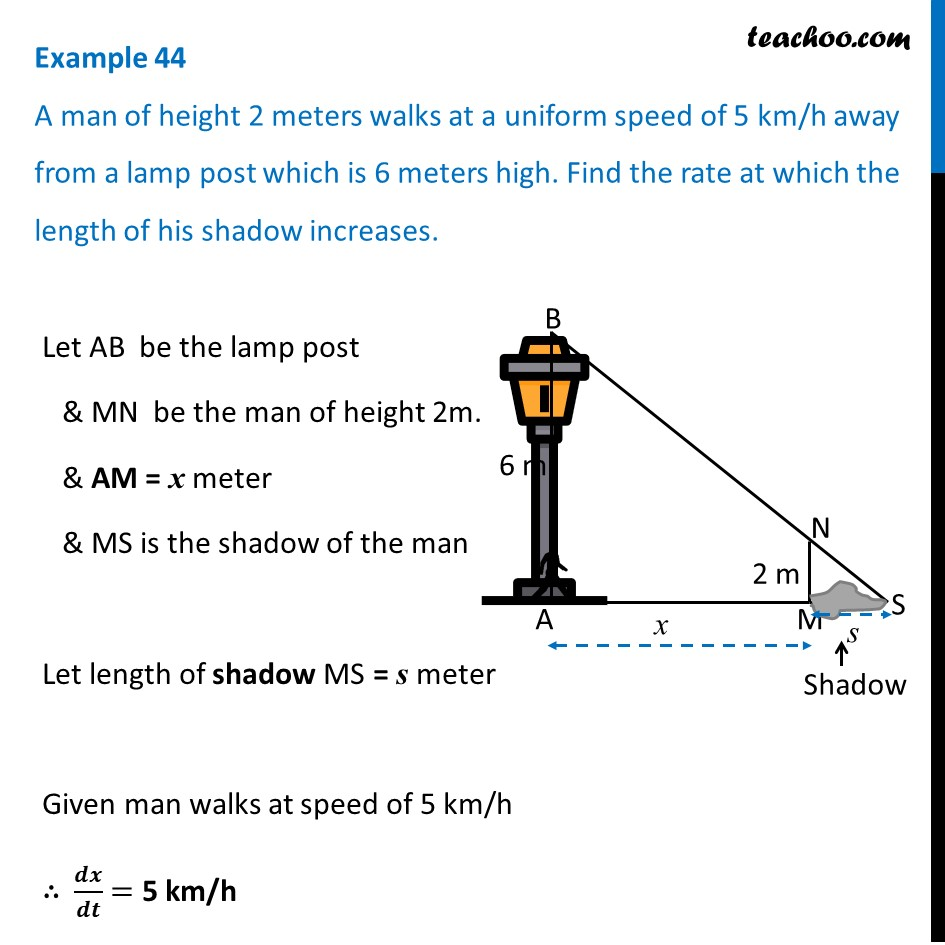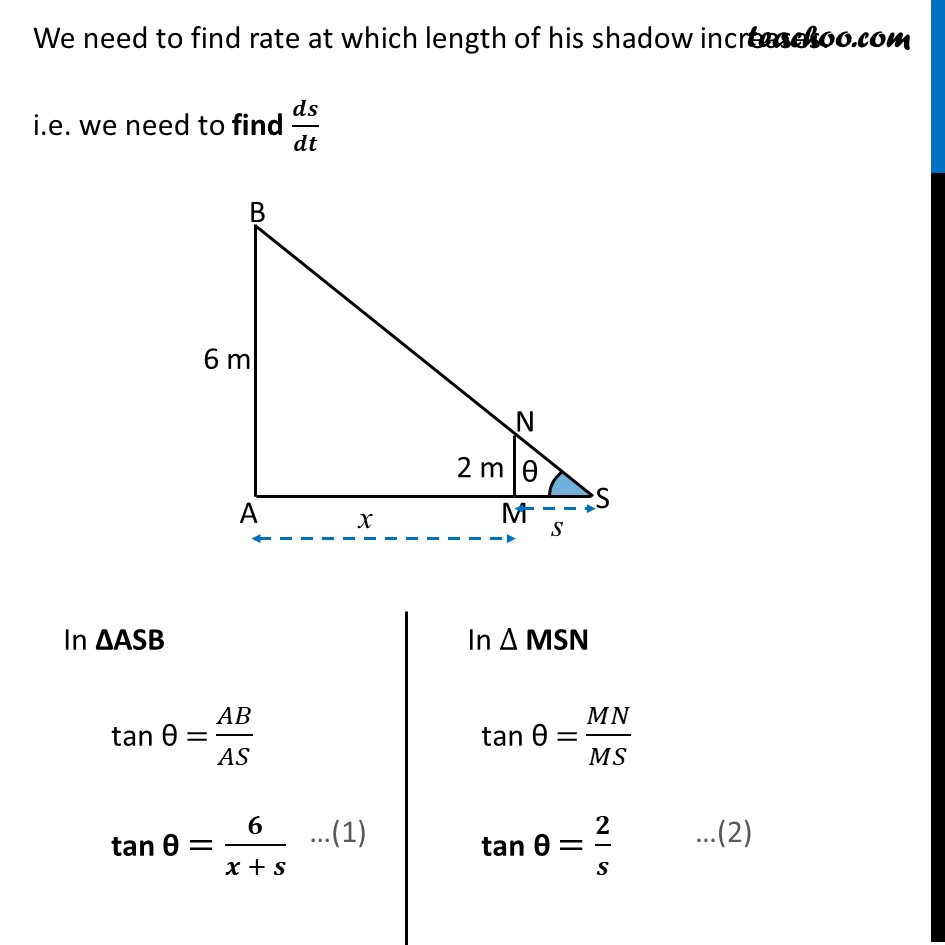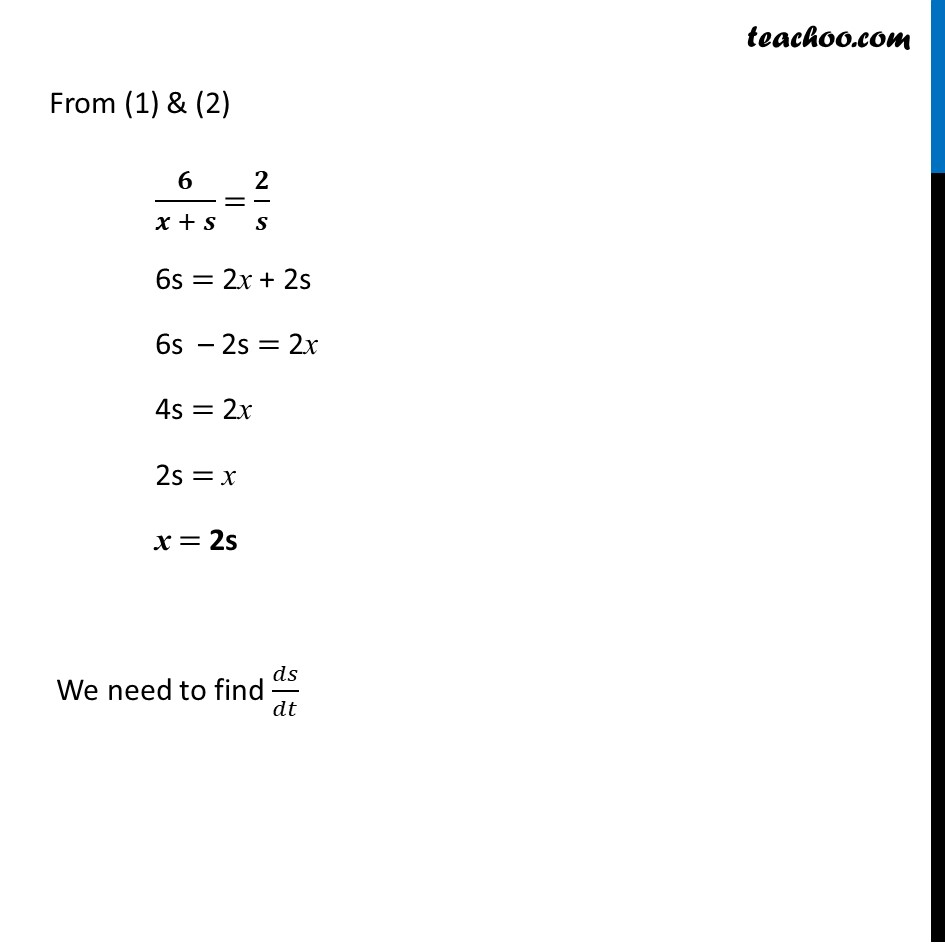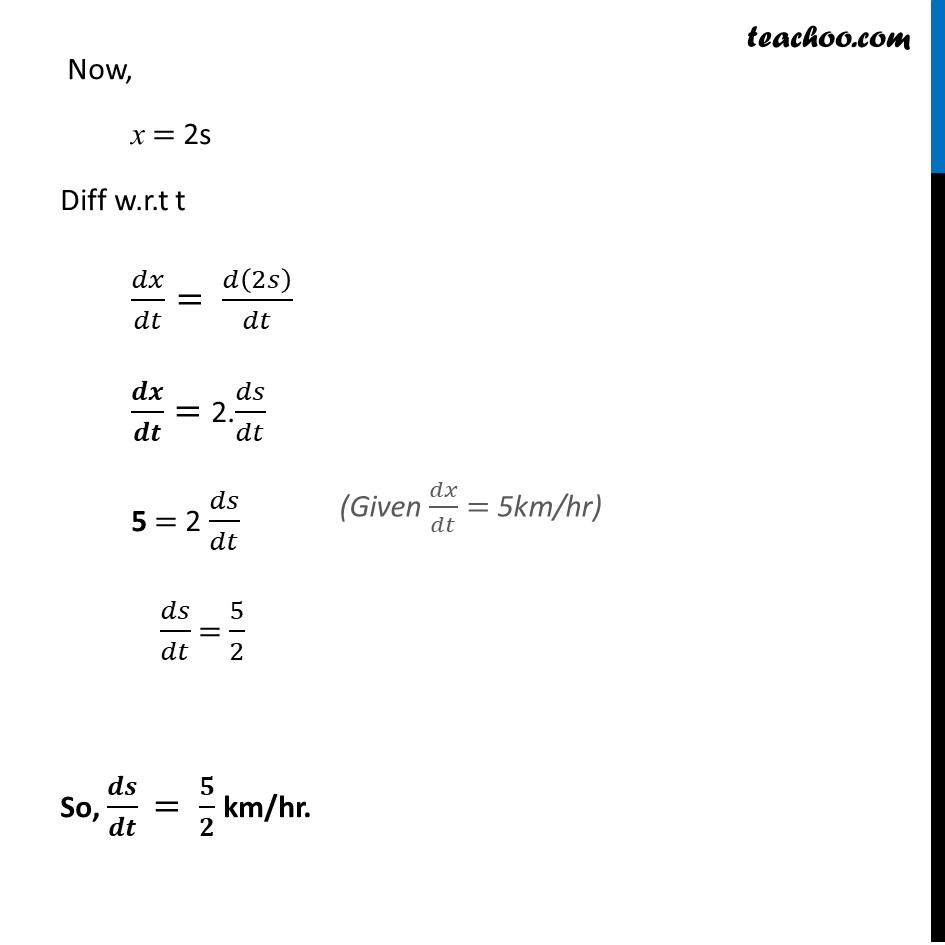Finding rate of change

Chapter 6 Class 12 Application of Derivatives (Term 1)
Concept wise### Transcript

Example 44 A man of height 2 meters walks at a uniform speed of 5 km/h away from a lamp post which is 6 meters high. Find the rate at which the length of his shadow increases.Let AB be the lamp post & MN be the man of height 2m. & AM = x meter & MS is the shadow of the man Let length of shadow MS = s meter Given man walks at speed of 5 km/h ∴ 𝒅𝒙/𝒅𝒕 = 5 km/h We need to find rate at which length of his shadow increases. i.e. we need to find 𝒅𝒔/𝒅𝒕 In ΔASB tan θ = 𝐴𝐵/𝐴𝑆 tan θ =𝟔/(𝒙 + 𝒔) In ∆ MSN tan θ = 𝑀𝑁/𝑀𝑆 tan θ =𝟐/𝒔 From (1) & (2) 𝟔/(𝒙 + 𝒔) = 𝟐/𝒔 6s = 2x + 2s 6s – 2s = 2x 4s = 2x 2s = x x = 2s We need to find 𝑑𝑠/𝑑𝑡 Now, x = 2s Diff w.r.t t 𝑑𝑥/𝑑𝑡= 𝑑(2𝑠)/𝑑𝑡 𝒅𝒙/𝒅𝒕= 2.𝑑𝑠/𝑑𝑡 5 = 2 𝑑𝑠/𝑑𝑡 𝑑𝑠/𝑑𝑡 = 5/2 So, 𝒅𝒔/𝒅𝒕 = 𝟓/𝟐 km/hr. (Given 𝑑𝑥/𝑑𝑡 = 5km/hr)